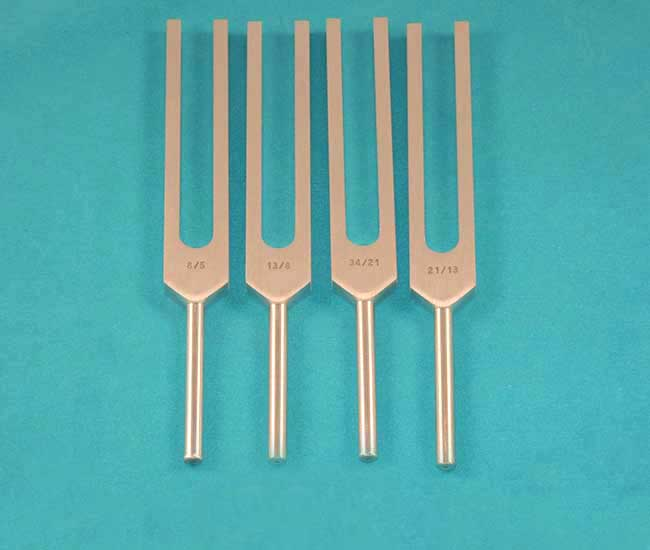Tuning Forks - Singing Bowls - Vibrational Healing Tools For Transformation
Home
>
Tuning Forks
>\$
80
Product Information

There are 8 Fibonacci Forks in the complete set, represented by the ratios derived in the Fibonacci number sequence. The sequence starts with 1, and continues with (1+1)= 2, (2+1)= 3, (3+2)= 5, (5+3)= 8, and so on. The last two numbers in the sequence combine to produce the next number... 1, 1, 2, 3, 5, 8, 13, 21, 34, etc. The first Fibonacci tuning fork is labeled 1/1, followed by 2/1, 3/2, 5/3, 8/5, 13/8, 21/13, and 34/21. Spiraling Intervals are created by using the reference fork (1/1) in combination with any other Fibonacci ratio fork. For example, to create the interval 8/5 use the 8/5 tuning fork with the 1/1 tuning fork. It is also possible to use two or more of any of the tuning forks in the set to create micro partial intervals.

If you already own the STD Harmonic Spectrum Set, it contains 4 of the 8 Fibonacci Forks: C-256 (1/1), C-512 (2/1), G-384 (3/2), and A-426.7 (5/3). With the addition of these 4 Add-On forks 8/5, 13/8, 21/13, and 34/21, you can make a complete Fibonacci Set.

All of our high quality tuning forks are Made in the USA. Each fork is triple tuned for accuracy of +/- .25% Hz. A protective velvet pouch is included.

### Standard or Weighted?

If you're unsure about the different uses for standard and weighted tuning forks, we're here to help!